Select Page

## SA Test

(Instructions)

In this test, there are drawing of all kinds of figure, and you have to imagine them in different positions. In each row, left of the vertical line, there is one figure-called "Sample Figure". The Sample Figure is followed by four "Test Figures". Some of the Test Figures will be the same as the Sample Figure, but just turned "around". For example:

S= the same (only turned around)

SAMPLE FIGURETEST FIGURES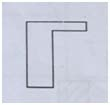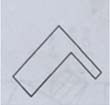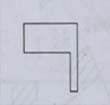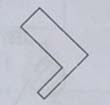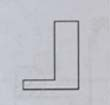Note: The Test Figures are the Sample Figure turned around.

Some of the Test Figures will be reversed from the Sample Figure; that is, they will look like the Sample Figure but would look as if it were turned "over". For example, here are some Test Figures that are reversed. In some cases, the Test Figure will be turned “over” and turned “around”; other times it will just be turned over.

R= Reversed (or turned over)

SAMPLE FIGURETEST FIGURES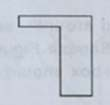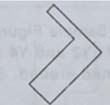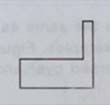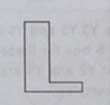Here the Test Figures looks like the Sample Figures turned over, or turned over and then turned around.

If a Test Figure is the same as the Sample Figure, click the S box for that number. If the Test Figure is the reversed from the Sample Figure, click the R box for that number.

The following examples are like those you will find ahead. The answers for the examples are pre-clicked for you. Look at each one of the six. Test Figures- X1 to X6 below, decide whether it is the same (S) or reversed from (R) the Sample Figure, and then check your answers with those that are pre-clicked. Remember, some of the six will be the same (S), but turned around. Others will be reversed (R) or turned over and, in some cases, then turned around.

SAMPLE FIGURETEST FIGURES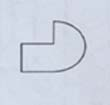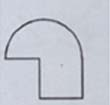S R
X1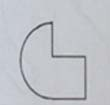S R
X2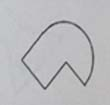S R
X3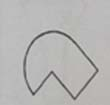S R
X4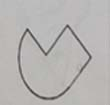S R
X5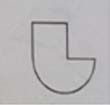S R
X6

Note that Test Figures X1, X4 and X6 are the same (S) as the Test Figures. They are simply the Sample Figure turned around. Test Figures X2, X3 and X5 are reversed from the Sample Figure. In X2, the Sample Figure was only turned over and in X3 and X5, it also turned around.

So you see the S (Same) box clicked for X1, X4 and X6, and the R (Reversed) box clicked for X2, X3 and X5. Now try another example, marking your answers in the Example Y1 - Y6 box. Work as quickly as you can.

SAMPLE FIGURETEST FIGURES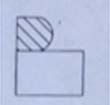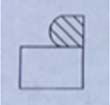S R
Y1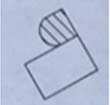S R
Y2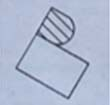S R
Y3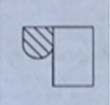S R
Y4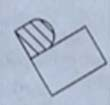S R
Y5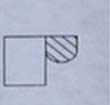S R
Y6

Test Figures Y3, Y5 and Y6 are the same as the Sample Figure (turned around), so you should have filled in the S box for these examples. Figures Y1, Y2 and Y4 are the Sample Figure turned over and you see that Y2 and Y4 are turned over and turned around. So the R box should have been filled in for these examples.

In the test, there will be one Sample Figure and six Test Figures in each row, as in the examples. After doing the six items (1-6) in the first row, go to the next row and do the Test Figures. Continue to work each row until you finish.

Be sure to mark S or R for each of the six Test Figures in each row. You will have to work very quickly to finish. If you are not sure of the right answer for an item, mark the choice that is your best guess.

When you are ready click the button below to proceed